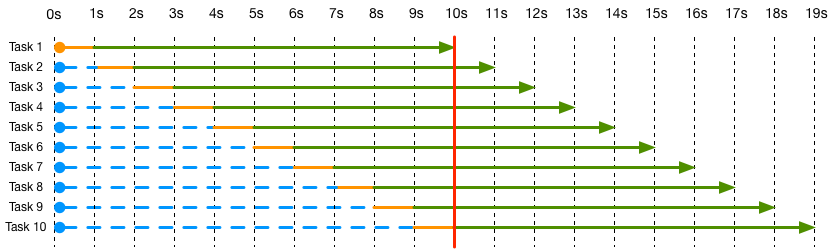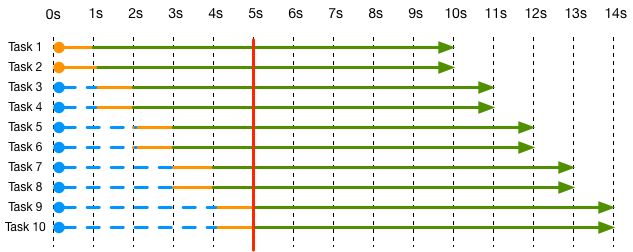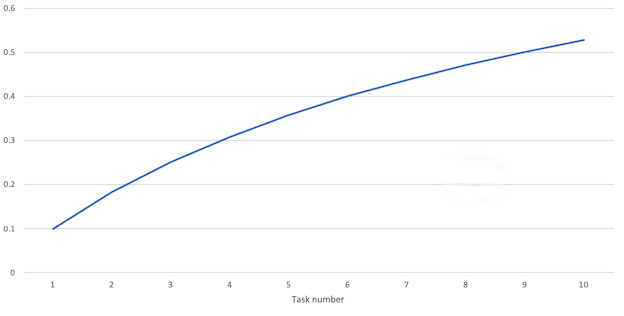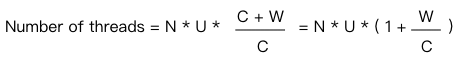# How to estimate throughput and thread pool size

## Estimating Throughput

Now there is a task which execution time is divided into 2 parts, the first part does math and the second part waits for IO. these two parts are called compute operation and wait operation.

So now we need to estimate the peak throughput that can be achieved by executing this task with the CPU at full power.

Then we need to know how much time it takes to execute this task, how much time is spent on the computation part and how much time is spent on the wait part.

Assuming that the computation part of the task takes 1 second and the waiting part takes 9 seconds, and that 10 threads are opened to execute 10 tasks, the following execution graph can be obtained with a single-core CPU.The blue line in the graph is the waiting schedule, the orange line is the execution of the computation task, and the green line is the time spent by the CPU waiting.

You can benefit from having 10 threads open, each task can use the waiting time of other tasks to perform its own computational operations, while keeping the CPU always busy, i.e. 100% utilization. This also tells you that even if you open 11 threads, you won’t get more benefits.

In reality, the OS will run these 10 threads alternately, see the red line in the diagram, and the OS can schedule the computation tasks of these 10 tasks as many times as it wants within this range. If the overhead of thread scheduling is removed, the total time spent is still equal to 10 seconds.

The throughput of this diagram becomes obvious: ``The throughput of

``````throughput = 10 tasks / (10 * computing time + wait time)
= 10 tasks / (10 * 1s + 9s)
= 10 / 19s = 0.526 tasks/s
``````

What if we now have a dual-core CPU?You can see that because there are 2 CPU cores, the computational tasks can overlap and thus the time spent is halved and the throughput is

``````throughput = 10 tasks / (10 * computing time / 2 + wait time)
= 10 tasks / (10 * 1s / 2 + 9s)
= 10 / 14s = 0.7142 tasks/s
``````

So to summarize the throughput calculation formula.

• Throughput: throughput.
• C: Number of CPUs. Can have a decimal number, such as 0.5, which means only half of the computing power is provided.
• Tc: time spent by task computation.
• Tw: the time spent by task waiting.
• E: time consumed for the last task to finish.C=1 in the formula means that the CPU is working at 100% of full speed, if C=0.5 then it means that the CPU has 50% of idle time, if C=2 then it means that two CPUs are started to run at full speed.

You can see that to increase the throughput there are.

• Increase C, which will be discussed below.
• Decrease Tc
• Decrease Tw

The general idea is to use more CPU cores to make the task run in less time.

Maybe you think you can also improve throughput by increasing Tn, as in the following figure.You can see that the throughput goes up with the number of tasks, so will it always be longer? The answer is no, as Tn gets bigger and bigger, Tn * Tc / C will also get bigger and bigger, then you can ignore Tw and the formula becomes C / Tc, which is the theoretical upper bound on throughput, and increasing Tn will only converge to this value infinitely.

So the question is, how do you know how many threads to open to get the CPU to reach the target utilization?

This depends on the following equation.

• N: Number of CPUs.
• U: CPU utilization, 0.1 means 10%, 1 means 100%.
• C: time used CPU.
• W: Waiting time.Note: N * U in this formula = C in the throughput formula.

If U = 1 (100% utilization), it is the ratio of W to C that determines the number of threads, when W is higher then more threads are needed, when W = 0, only the same number of threads as N is needed.

This formula also tells us that opening more threads will not bring additional benefits but will cause the opposite effect (increased thread scheduling overhead), so in practice we use thread pools with upper bounds.

And when actually doing performance tuning, the thread pool size is adjusted around the calculated number to achieve the best results.